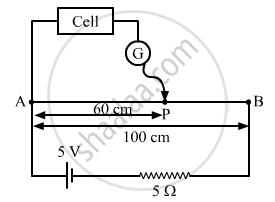# A Potentiometer Wire of Length 1.0 M Has a Resistance of 15 ω. It is Connected to a 5 V Battery in Series with a Resistance of 5 ω. Determine the Emf of the Primary Cell Which Gives a Balance - Physics

A potentiometer wire of length 1.0 m has a resistance of 15 Ω. It is connected to a 5 V battery in series with a resistance of 5 Ω. Determine the emf of the primary cell which gives a balance point at 60 cm.

#### SolutionFrom the figure:
Total resistance of the circuit , R = (RAB + 5) Ω = 20 Ω
Current in the circuit,

$i = \frac{V}{R} = \frac{5}{20} A$

∴ Voltage across AB, VAB = i.RAB = 3.75 V
The emf of the cell connected as above is given by:

$e = \frac{l}{L} V_0$

Here, l = 60 cm (balance point)
L = 1 m = 100 cm (total length of the wire)

$\therefore e = \frac{60}{100}\left( 3 . 75 \right) = 2 . 25 V$

Concept: Cells, Emf, Internal Resistance
Is there an error in this question or solution?